# Confusion Matrix: How To Use It & Interpret Results [Examples]

A confusion matrix is used for evaluating the performance of a machine learning model. Learn how to interpret it to assess your model's accuracy.11
September 13, 2022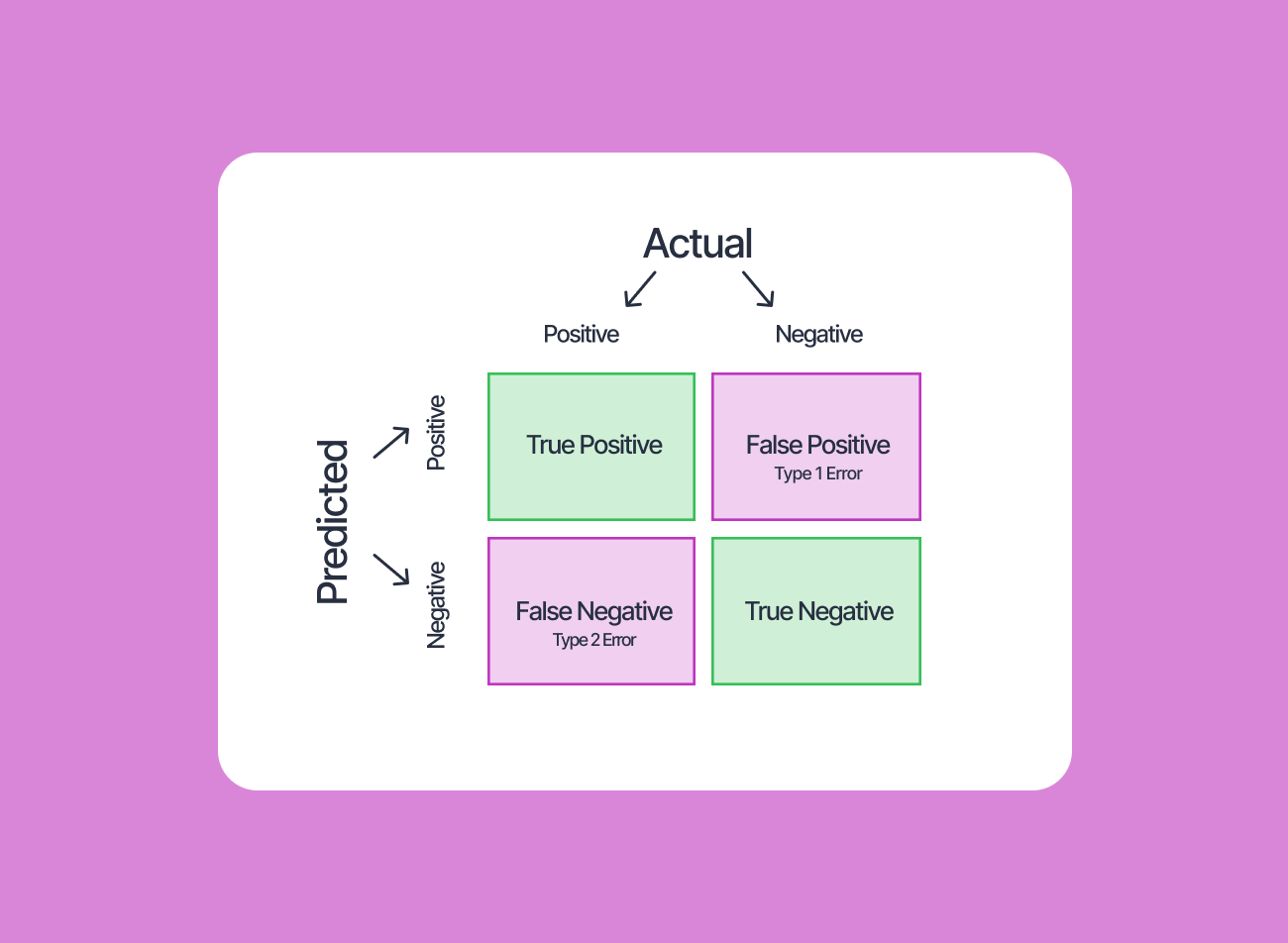Deep Learning is now the most popular technique for solving any Computer Vision task—from image classification and segmentation to 3D scene reconstruction or neural rendering.

But how do you know if a deep model is performing well? We can use “accuracy” as an evaluation metric, right?

So, what does "accuracy" really tell us? It tells us how many correct predictions a model will make when given 100 samples.

Yet, that is not enough information to analyze a model's performance. What if the prediction task consists of 5 different classes of samples, and the model constantly makes wrong predictions on one of these classes, e.g., class-4?

The model might seem to have an accuracy of 90% if the test set contains an imbalanced number of samples (i.e., samples from class-4 might be few), but still, it is not a good performer.

This is where confusion matrices come in. A confusion matrix is a more comprehensive mode of evaluation that provides more insight to the ML engineer about their model's performance.

Solve any video or image labeling task 10x faster and with 10x less manual work.

Don't start empty-handed. Explore our repository of 500+ open datasets and test-drive V7's tools.

Ready to streamline AI product deployment right away? Check out:

## What is a Confusion Matrix?

A confusion matrix, as the name suggests, is a matrix of numbers that tell us where a model gets confused. It is a class-wise distribution of the predictive performance of a classification model—that is, the confusion matrix is an organized way of mapping the predictions to the original classes to which the data belong.

This also implies that confusion matrices can only be used when the output distribution is known, i.e., in supervised learning frameworks.

The confusion matrix not only allows the calculation of the accuracy of a classifier, be it the global or the class-wise accuracy, but also helps compute other important metrics that developers often use to evaluate their models.

A confusion matrix computed for the same test set of a dataset, but using different classifiers, can also help compare their relative strengths and weaknesses and draw an inference about how they can be combined (ensemble learning) to obtain the optimal performance.

Although the concepts for confusion matrices are similar regardless of the number of classes in the dataset, it is helpful to first understand the confusion matrix for a binary class dataset and then interpolate those ideas to datasets with three or more classes. Let us dive into that next.

## Confusion Matrix for Binary Classes

A binary class dataset is one that consists of just two distinct categories of data.

These two categories can be named the “positive” and “negative” for the sake of simplicity.

Suppose we have a binary class imbalanced dataset consisting of 60 samples in the positive class and 40 samples in the negative class of the test set, which we use to evaluate a machine learning model.

Now, to fully understand the confusion matrix for this binary class classification problem, we first need to get familiar with the following terms:

• True Positive (TP) refers to a sample belonging to the positive class being classified correctly.
• True Negative (TN) refers to a sample belonging to the negative class being classified correctly.
• False Positive (FP) refers to a sample belonging to the negative class but being classified wrongly as belonging to the positive class.
• False Negative (FN) refers to a sample belonging to the positive class but being classified wrongly as belonging to the negative class.

An example of the confusion matrix we may obtain with the trained model is shown above for this example dataset. This gives us a lot more information than just the accuracy of the model.

Adding the numbers in the first column, we see that the total samples in the positive class are 45+15=60. Similarly, adding the numbers in the second column gives us the number of samples in the negative class, which is 40 in this case. The sum of the numbers in all the boxes gives the total number of samples evaluated. Further, the correct classifications are the diagonal elements of the matrix—45 for the positive class and 32 for the negative class.

Now, 15 samples (bottom-left box) that were expected to be of the positive class were classified as the negative class by the model. So it is called “False Negatives” because the model predicted “negative,” which was wrong. Similarly, 8 samples (top-right box) were expected to be of negative class but were classified as “positive” by the model. They are thus called “False Positives.” We can evaluate the model more closely using these four different numbers from the matrix.

In general, we can get the following quantitative evaluation metrics from this binary class confusion matrix:

1. Accuracy. The number of samples correctly classified out of all the samples present in the test set.

1. Precision (for the positive class). The number of samples actually belonging to the positive class out of all the samples that were predicted to be of the positive class by the model.

1. Recall (for the positive class). The number of samples predicted correctly to be belonging to the positive class out of all the samples that actually belong to the positive class.

1. F1-Score (for the positive class). The harmonic mean of the precision and recall scores obtained for the positive class.
1. Specificity. The number of samples predicted correctly to be in the negative class out of all the samples in the dataset that actually belong to the negative class.

## Confusion Matrix for Multiple Classes

The concept of the multi-class confusion matrix is similar to the binary-class matrix. The columns represent the original or expected class distribution, and the rows represent the predicted or output distribution by the classifier.

Let us elaborate on the features of the multi-class confusion matrix with an example. Suppose we have the test set (consisting of 191 total samples) of a dataset with the following distribution:

The confusion matrix obtained by training a classifier and evaluating the trained model on this test set is shown below. Let that matrix be called “M,” and each element in the matrix be denoted by “M_ij,” where “i” is the row number (predicted class), and “j” is the column number (expected class), e.g., M_11=52, M_42=1.

This confusion matrix gives a lot of information about the model’s performance:

• As usual, the diagonal elements are the correctly predicted samples. A total of 145 samples were correctly predicted out of the total 191 samples. Thus, the overall accuracy is 75.92%.
• M_24=0 implies that the model does not confuse samples originally belonging to class-4 with class-2, i.e., the classification boundary between classes 2 and 4 was learned well by the classifier.
• To improve the model’s performance, one should focus on the predictive results in class-3. A total of 18 samples (adding the numbers in the red boxes of column 3) were misclassified by the classifier, which is the highest misclassification rate among all the classes. Accuracy in prediction for class-3 is, thus, 58.14% only.

The confusion matrix can be converted into a one-vs-all type matrix (binary-class confusion matrix) for calculating class-wise metrics like accuracy, precision, recall, etc.

Converting the matrix to a one-vs-all matrix for class-1 of the data looks like as shown below. Here, the positive class refers to class-1, and the negative class refers to “NOT class-1”. Now, the formulae for the binary-class confusion matrices can be used for calculating the class-wise metrics.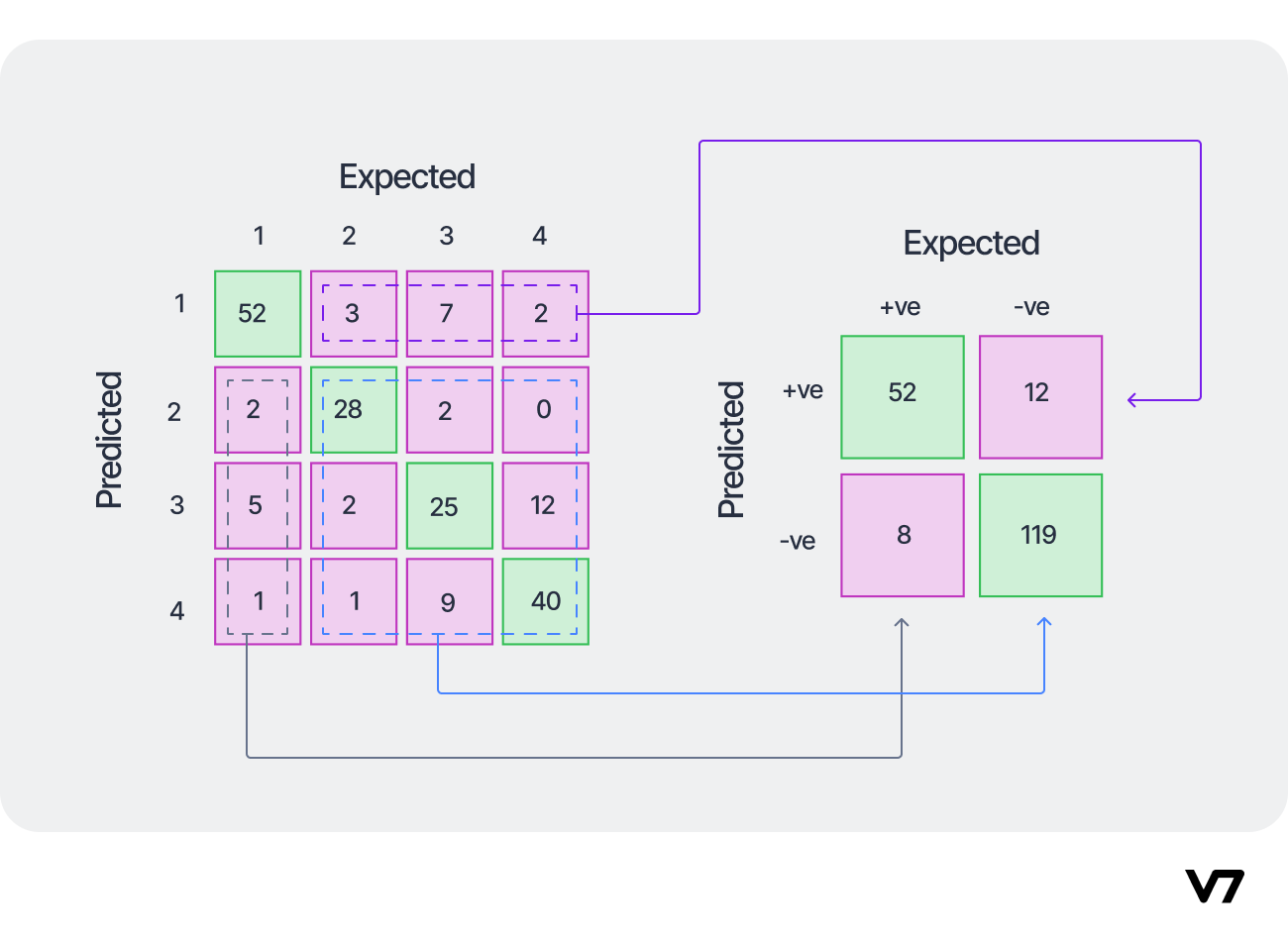Converting a multi-class confusion matrix to a one-vs-all (for class-1) matrix. Image by the author.

Similarly, for class-2, the converted one-vs-all confusion matrix will look like the following: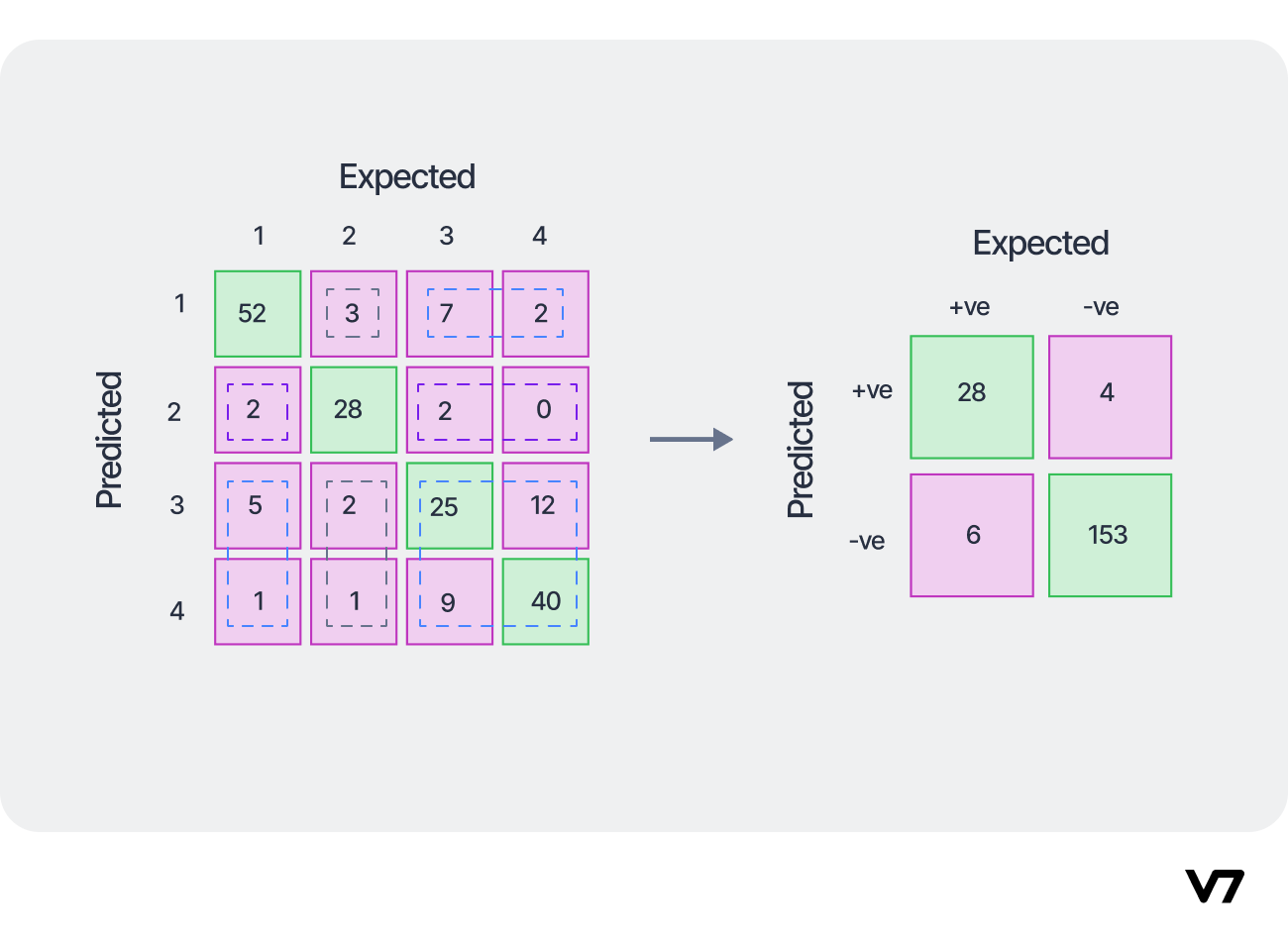Converting a multi-class confusion matrix to a one-vs-all (for class-2) matrix. Image by the author.

Using this concept, we can calculate the class-wise accuracy, precision, recall, and f1-scores and tabulate the results:

In addition to these, two more global metrics can be calculated for evaluating the model’s performance over the entire dataset. These metrics are variations of the F1-Score we calculated here. Let us look into them next.

### Micro F1-Score

The micro-averaged f1-score is a global metric that is calculated by considering the net TP, i.e., the sum of the class-wise TP (from the respective one-vs-all matrices), net FP, and net FN. These are obtained to be the following:

Net TP = 52+28+25+40 = 145
Net FP = (3+7+2)+(2+2+0)+(5+2+12)+(1+1+9) = 46
Net FN = (2+5+1)+(3+2+1)+(7+2+9)+(2+0+12) = 46

Note that for every confusion matrix, the net FP and net FN will have the same value. Thus, the micro precision and micro recall can be calculated as:

Micro Precision = Net TP/(Net TP+Net FP) = 145/(145+46) = 75.92%
Micro Recall = Net TP/(Net TP+Net FN) = 75.92%

Thus, Micro F-1 = Harmonic Mean of Micro Precision and Micro Recall = 75.92%.

Since all the measures are global, we get:
Micro Precision = Micro Recall = Micro F1-Score = Accuracy = 75.92%

### Macro F1-Score

The macro-averaged scores are calculated for each class individually, and then the unweighted mean of the measures is calculated to calculate the net global score. For the example we have been using, the scores are obtained as the following:

The unweighted means of the measures are obtained to be:

Macro Precision = 76.00%
Macro Recall = 75.31%
Macro F1-Score = 75.60%

### Weighted F1-Score

The weighted-average scores take a sample-weighted mean of the class-wise scores obtained. So, the weighted scores obtained are:

A Receiver Operating Characteristics (ROC) curve is a plot of the “true positive rate” with respect to the “false positive rate” at different threshold settings. ROC curves are usually defined for a binary classification model, although that can be extended to a multi-class setting, which we will see later.

The definition of the true positive rate (TPR) coincides exactly with the sensitivity (or recall) parameter- as the number of samples belonging to the positive class of a dataset, being classified correctly by the predictive model. So the formula for computing the TPR simply,

The false positive rate (FP) is defined as the number of negative class samples predicted wrongly to be in the positive class (i.e., the False Positives), out of all the samples in the dataset that actually belong to the negative class. Mathematically it is represented as the following:

Note that mathematically, the FPR is the additive inverse of Specificity (as shown above). So both the TPR and FPR can be computed easily from our existing computations from the Confusion Matrix.

Now, what do we mean by “thresholds” in the context of ROC curves? Different thresholds represent the different possible classification boundaries of a model. Let us understand this with an example. Suppose we have a binary class dataset with 4 positive class samples and 6 negative class samples, and the model decision boundary is as shown by the blue line in case (A) below. The RIGHT side of the decision boundary depicts the positive class, and the LEFT side depicts the negative class.

Now, this decision boundary threshold can be changed to arrive at case (B), where the precision is 100% (but recall is 50%), or to case (C) where the recall is 100% (but precision is 50%). The corresponding confusion matrices are shown. The TPR and FPR values for these three scenarios with the different thresholds are thus as shown below.

##### 💡Read More:Precision vs. Recall: Differences, Use Cases & Evaluation

Using these values, the ROC curve can be plotted. An example of a ROC curve for a binary classification problem (with randomly generated samples) is shown below.

A learner that makes random predictions is called a “No Skill” classifier. For a class-balanced dataset, the class-wise probabilities will be 50%. It acts as a reference line for the plot of the precision-recall curve. A perfect learner is one which classifies every sample correctly, and it also acts as a reference line for the ROC plot.

A real-life classifier will have a plot somewhere in between these two reference lines. The more a ROC of a learner is shifted towards the (0.0, 1.0) point (i.e., towards the perfect learner curve), the better is its predictive performance across all thresholds.

Another important metric that measures the overall performance of a classifier is the “Area Under ROC” or AUROC (or just AUC) value. As the name suggests, it is simply the area measured under the ROC curve. A higher value of AUC represents a better classifier. The AUC of the practical learner above is 90% which is a good score. The AUC of the no skill learner is 50% and that for the perfect learner is 100%.

For multi-class datasets, the ROC curves are plotted by dissolving the confusion matrix into one-vs-all matrices, which we have already seen how to do. This paper, for example, addressed the cervical cancer detection problem and utilized multi-class ROC curves to get a deep dive analysis of their model performance.

Train ML models 10x faster

Turn labeled data into models. Develop production-ready AI in hours with just a few clicks.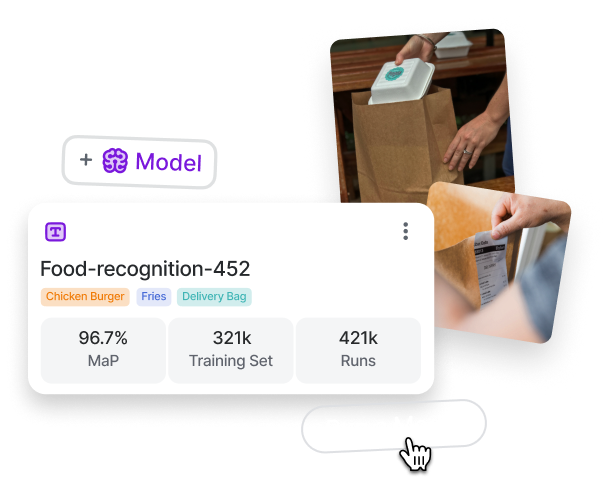## Tools for Computing a Confusion Matrix

Python can be easily used to compute the confusion matrix and the micro, macro, and weighted metrics we discussed above.

The scikit-learn package of Python contains all these tools. For example, using the function “confusion_matrix” and entering the true label distribution and predicted label distribution (in that order) as the arguments, one can get the confusion matrix as follows:

Note that the confusion matrix printed here is the transposed version of what we have been using as an example throughout the article. That is, in this Python version, rows represent the expected class labels, and columns represent the predicted class labels. The evaluation metrics and the concepts explained are still valid.

In other words, for a binary confusion matrix, the TP, TN, FP, and FN will look like this: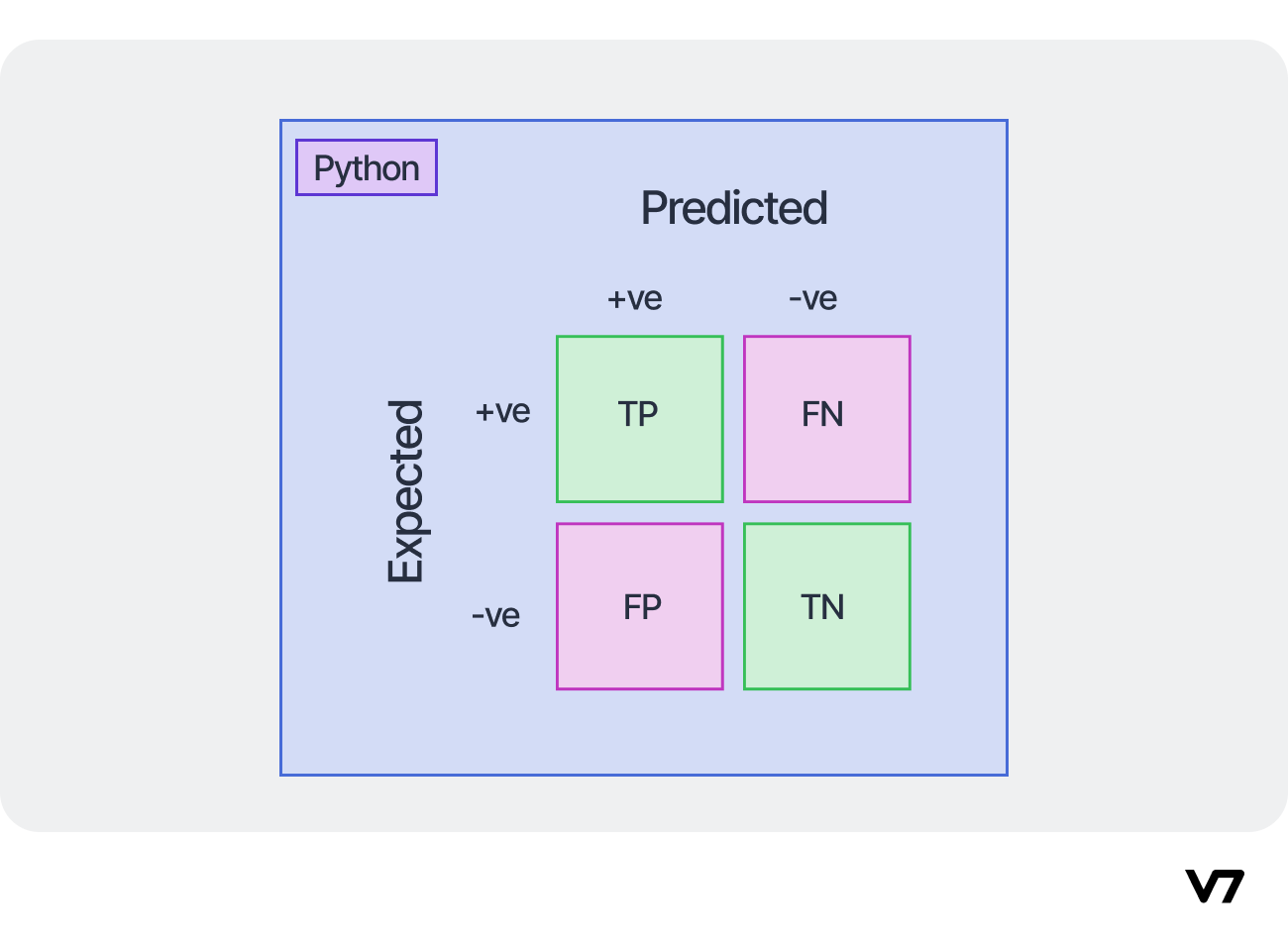Representation of a confusion matrix in Python. Image by the author.

In Python, we also have the option to output the confusion matrix as a heatmap using the ConfusionMatrixDisplay function, visually showcasing which cases have a more significant error rate. However, to use the heatmap, it is wiser to use a normalized confusion matrix because the dataset may be imbalanced. Thus, the representation in such cases might not be accurate. The confusion matrices (both un-normalized and normalized) for the multi-class data example we have been following are shown below.

Since the dataset is unbalanced, the un-normalized confusion matrix does not give an accurate representation of the heatmap. For example, M_22=28, which is shown as a low-intensity heatmap in the un-normalized matrix, where actually it represents 82.35% accuracy for class-2 (which has only 34 samples), which is decently high. This trend has been correctly captured in the normalized matrix, where a high intensity has been portrayed for M_22. Thus, for generating heat maps, a normalized confusion matrix is desired.

The micro, macro, and weighted averaged precision, recall, and f1-scores can be obtained using the “classification_report” function of scikit-learn in Python, again by using the true label distribution and predicted label distribution (in that order) as the arguments. The results obtained will look like as shown:

Here, the column “support” represents the number of samples that were present in each class of the test set.

Plotting the ROC curve for a binary-class classification problem in Python is simple, and involves using the “roc_curve” function of scikit-learn. The true labels of the samples and the prediction probability scores (not the predicted class labels.) are taken as the input in the function, to return the FPR, TPR and the threshold values. An example is shown below.

The roc_curve function outputs the discrete coordinates for the curve. The “matplotlib.pyplot” function of Python is used here to actually plot the curve using the obtained coordinates in a GUI.

Plotting the ROC curves for a multi-class classification problem takes a few more steps, which we will not cover in this article. However, the Python implementation of multi-class ROC is explained here in detail.

Computing the area under curve value takes just one line of code in Python using the “roc_auc_score” function of scikit-learn. It takes as input again, the true labels and the prediction probabilities and returns the AUROC or AUC value as shown below.

## Confusion Matrix—Example or Recent Application

A crucial example where a confusion matrix can aid an application-specific model training is COVID-19 detection

COVID-19, as we all know, is infamous for spreading quickly. So, for a model that classifies medical images (lung X-rays or CT-Scans) into “COVID positive” and “COVID negative” classes, we would want the False Negative rate to be the lowest. That is, we do not want a COVID-positive case to be classified as COVID-negative because it increases the risk of COVID spread from that patient.

After all, only COVID-positive patients can be quarantined to prevent the spread of the disease. This has been explored in this paper.

## Key Takeaways

The success or failure of machine learning models depends on how we evaluate them. Detailed model analysis is essential for drawing a fair conclusion about its performance.

Although most methods in the literature only report the accuracy of classifiers, it is not enough to judge whether the model really learned the distinct class boundaries of the dataset.

The confusion matrix is a succinct and organized way of getting deeper information about a classifier which is computed by mapping the expected (or true) outcomes to the predicted outcomes of a model.

Along with classification accuracy, it also enables the computation of metrics like precision, recall (or sensitivity), and f1-score, both at the class-wise and global levels, which allows ML engineers to identify where the model needs to improve and take appropriate corrective measures.

Looking for other resources? Explore other machine learning and computer vision subjects:Rohit Kundu is a Ph.D. student in the Electrical and Computer Engineering department of the University of California, Riverside. He is a researcher in the Vision-Language domain of AI and published several papers in top-tier conferences and notable peer-reviewed journals.

“Collecting user feedback and using human-in-the-loop methods for quality control are crucial for improving Al models over time and ensuring their reliability and safety. Capturing data on the inputs, outputs, user actions, and corrections can help filter and refine the dataset for fine-tuning and developing secure ML solutions.”Name
Company
GUIDE
Building AI-Powered Products: The Enterprise Guide

Building AI products? This guide breaks down the A to Z of delivering an AI success story.

Oops! Something went wrong while submitting the form.## Related articles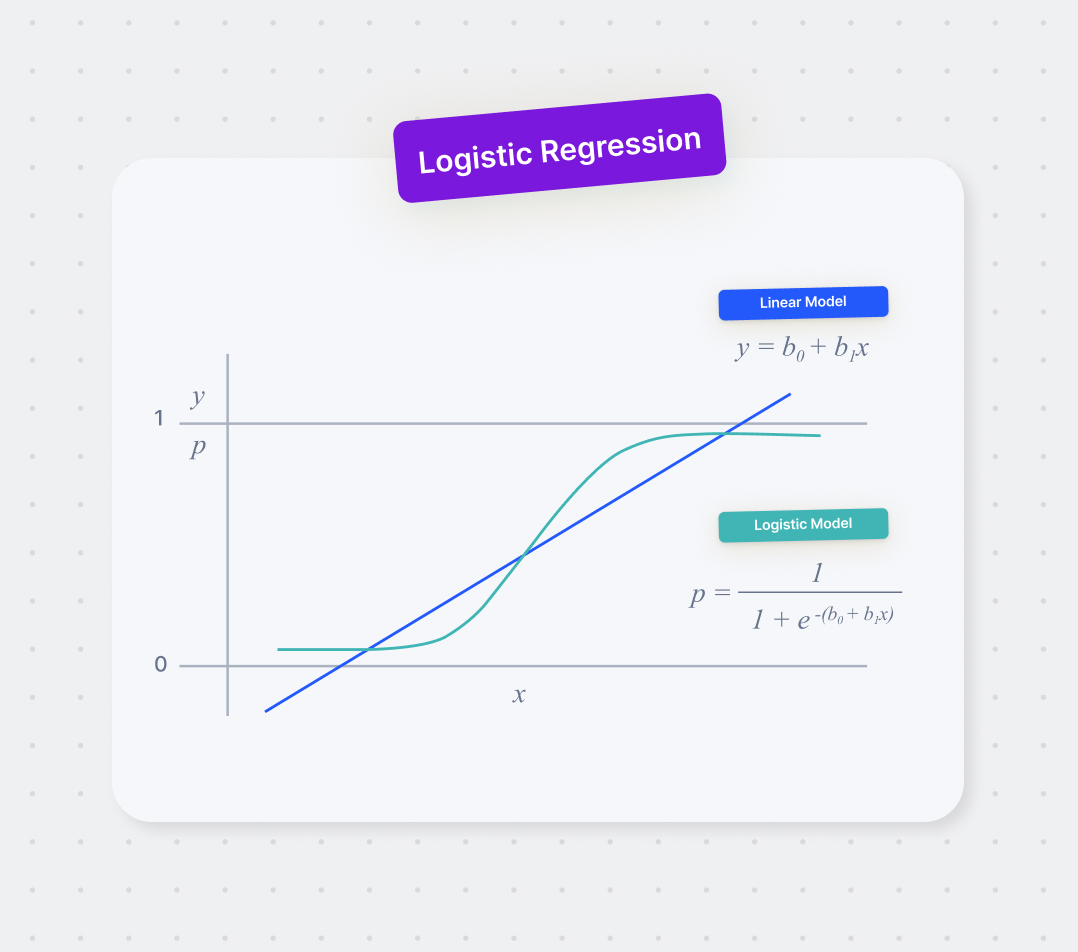Logistic Regression: Definition, Use Cases, Implementation
Deval Shah